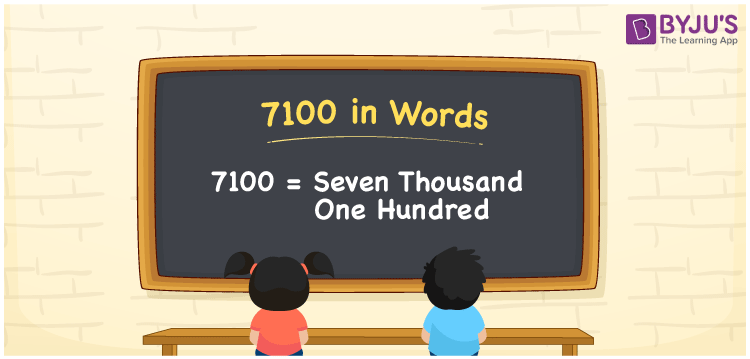# 7100 in Words

7100 in words is “Seven Thousand One Hundred”. The number 7100 is a cardinal number as it helps to count the objects or specify the values. The number 7100 can be converted into words using the place value system. If a library contains 7100 books, it can be written as “A library contains seven thousand one hundred books”. In this article, let us discuss the spelling of 7100 in English words with its procedure in detail.

 7100 in Words: Seven Thousand One Hundred. Seven Thousand One Hundred in Numerical Form: 7100.

## 7100 in English Words

The number 7100 in English words is “Seven Thousand One Hundred”.## How to Write 7100 in Words?

Here is the place value table for the number 7100.

 Thousands Hundreds Tens Ones 7 1 0 0

The expanded form of 7100 is as follows:

= 7 × Thousand + 1 × Hundred + 0 × Ten + 0 × One

= 7 × 1000 + 1 × 100

= 7000 + 100

= 7100

= seven thousand one hundred

Hence, 7100 in words is seven thousand one hundred.

7100 in words – Seven thousand one hundred

Is 7100 an odd number? – No

Is 7100 an even number? – Yes

Is 7100 a perfect square number? – No

Is 7100 a perfect cube number? – No

Is 7100 a prime number? – No

Is 7100 a composite number? – Yes

## Frequently Asked Questions on 7100 in Words

### Write 7100 in words.

7100 in words is seven thousand one hundred.

### Simplify 7000 + 100, and express in words.

Simplifying 7000 + 100, we get 7100. Hence, 7100 in words is seven thousand one hundred.

### Is 7100 a composite number?

Yes, 7100 is a composite number.